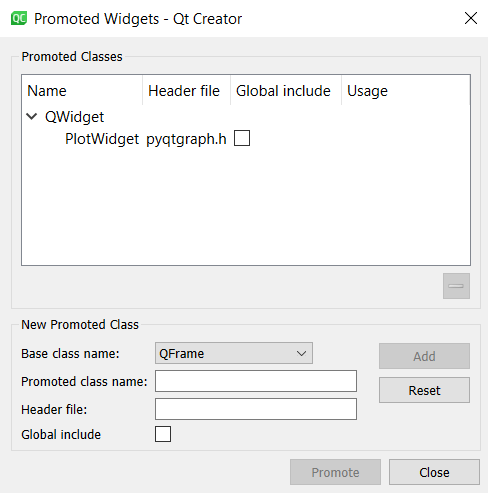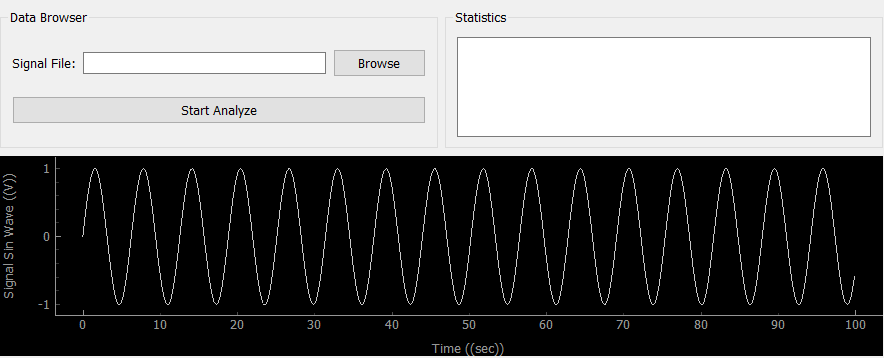GFG App
Open AppBrowser
Continue

# Add QT GUI to Python for plotting graphics

Qt framework (with QT Creator IDE) can be used to create a fancy interfaces for Python GUI application. Plotting graphics on a GUI is possible with pyqtgraph library.

Installing pyqtgraph –
There are several ways of installing pyqtgraph depending on your needs.

If you are using Anaconda you can install with:

```conda install -c anaconda pyqtgraph
```

Or with pip command:

```pip install pyqtgraph
```

Creation of plot widgets with QT Creator –

Add the buttons, text areas and other stuffs as usually done with QT Creator. To create a plot area you need to follow the steps:

1. Add widget to UI and give it a proper name like “widgetSignal”
2. Promote the widget to pyqtgraph1. In your python code call the UI you created with QT Creator.
2. Create a sin wave for plotting
3. Draw the graph on UI

 `from` `PyQt5 ``import` `QtWidgets, uic ` `import` `sys ` `import` `numpy as np ` ` `  `class` `MainWindow(QtWidgets.QMainWindow): ` `     `  `    ``def` `__init__(``self``, ``*``args, ``*``*``kwargs): ` `        ``super``(MainWindow, ``self``).__init__(``*``args, ``*``*``kwargs) ` `         ``# Load the UI Page ` `        ``self``. ui ``=` `uic.loadUi(``'mainwindow.ui'``, ``self``) ` `        ``# Create a sin wave ` `        ``x_time ``=` `np.arange(``0``, ``100``, ``0.1``); ` `        ``y_amplitude ``=` `np.sin(x_time) ` `         `  `        ``pltSignal ``=` `self``.widgetSignal ` `        ``pltSignal.clear() ` `        ``pltSignal.setLabel(``'left'``, ``'Signal Sin Wave'``, units ``=``'(V)'``) ` `        ``pltSignal.setLabel(``'bottom'``, ``'Time'``, units ``=``'(sec)'``) ` `        ``pltSignal.plot(x_time, y_amplitude, clear ``=` `True``) ` ` `  `        ``self``.ui.show() ` ` `  `def` `main(): ` `    ``app ``=` `QtWidgets.QApplication(sys.argv) ` `    ``window ``=` `MainWindow() ` `    ``sys.exit(app.exec_()) ` ` `  `if` `__name__ ``=``=` `'__main__'``: ` `    ``main()      `

Output:My Personal Notes arrow_drop_up